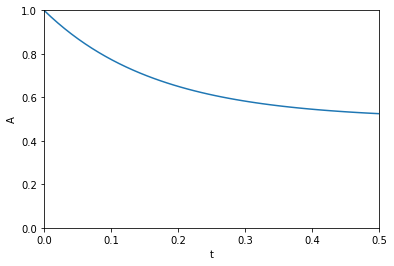## Sensitivity analysis using automatic differentiation in Python

| categories: | tags: | View Comments

This paper describes how sensitivity analysis requires access to the derivatives of a function. Say, for example we have a function describing the time evolution of the concentration of species A:

$$[A] = \frac{[A]_0}{k_1 + k_{-1}} (k_1 e^{(-(k_1 _ k_{-1})t)} + k_{-1})$$

The local sensitivity of the concentration of A to the parameters $$k1$$ and $$k_1$$ are defined as $$\frac{\partial A}{\partial k1}$$ and $$\frac{\partial A}{\partial k_1}$$. Our goal is to plot the sensitivity as a function of time. We could derive those derivatives, but we will use auto-differentiation instead through the autograd package. Here we import numpy from the autograd package and plot the function above.

import autograd.numpy as np

A0 = 1.0

def A(t, k1, k_1):
return A0 / (k1 + k_1) * (k1 * np.exp(-(k1 + k_1) * t) + k_1)

%matplotlib inline
import matplotlib.pyplot as plt

t = np.linspace(0, 0.5)

k1 = 3.0
k_1 = 3.0
plt.plot(t, A(t, k1, k_1))
plt.xlim([0, 0.5])
plt.ylim([0, 1])
plt.xlabel('t')
plt.ylabel('A')The figure above reproduces Fig. 1 from the paper referenced above. Next, we use autograd to get the derivatives. This is subtly different than our previous post. First, we need the derivative of the function with respect to the second and third arguments; the default is the first argument. Second, we want to evaluate this derivative at each time value. We use the jacobian function in autograd to get these. This is different than grad, which will sum up the derivatives at each time. That might be useful for regression, but not for sensitivity analysis. Finally, to reproduce Figure 2a, we plot the absolute value of the sensitivities.

from autograd import jacobian

plt.xlim([0, 0.5])
plt.ylim([0, 0.1])
plt.xlabel('t')
plt.legend(['$S_{k1}$', '$S_{k\_1}$'])That looks like the figure in the paper. To summarize the main takeaway, autograd enabled us to readily compute derivatives without having to derive them manually. There was a little subtlety in choosing jacobian over grad or elementwise_grad but once you know what these do, it seems reasonable. It is important to import the wrapped numpy first, to enable autograd to do its work. All the functions here are pretty standard, so everything worked out of the box. We should probably be using autograd, or something like it for more things in science!